Ch 7. Stress Analysis Multimedia Engineering Mechanics PlaneStress PrincipalStresses Mohr's Circlefor Stress Failure PressureVessels
 Chapter 1. Stress/Strain 2. Torsion 3. Beam Shr/Moment 4. Beam Stresses 5. Beam Deflections 6. Beam-Advanced 7. Stress Analysis 8. Strain Analysis 9. Columns Appendix Basic Math Units Basic Equations Sections Material Properties Structural Shapes Beam Equations Search eBooks Dynamics Fluids Math Mechanics Statics Thermodynamics Author(s): Kurt Gramoll ©Kurt GramollMECHANICS - THEORY

Thin-walled Pressure Vessels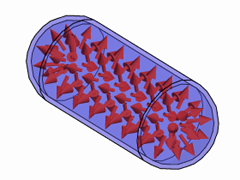Cylindrical Pressure Vessel with
Internal Pressure

Both cylinderical and spherical pressure vessels are common structures that are used ranging from large gas storage structures to small compressed air tanks in industrial equipment. In this section, only thin-walled pressure vessels will be analyzed.

A pressure vessel is assumed to be thin-walled if the wall thickness is less than 10% of the radius (r/t > 10). This condition assumes that the pressure load will be transfered into the shell as pure tension (or compression) without any bending. Thin-walled pressure vessels are also known as shell structures and are efficient storage structures.

If the outside pressure is greater than the inside pressure, the shell could also fail due to buckling. This is an advanced topic and is not considered in this section.

Cylindrical Pressure Vessels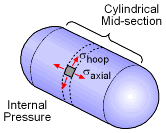Cylindrical Vessels will Expierence
Both Hoop and Axial Stress in
the Mid-section

Only the middle cylindrical section of a cylinder pressure vessel is examined in this section. The joint between the end caps and the mid-section will have complex stresses that are beyond the discussion in this chapter.

In the mid-section, the pressure will cause the vessel to expand or strain in only the axial (or longitudinal) and the hoop (or circumferential) directions. There will be no twisting or shear strains. Thus, there will only be the hoop stress, σh and the axial stress, σa. as shown in the diagram at the left.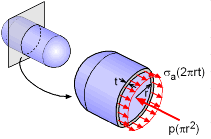Cross Section Cut of
Cylindrical Vessel

Pressure vessels can be analyzed by cutting them into two sections, and then equating the pressure load at the cut with the stress load in the thin walls. In the axial direction, the axial pressure from the discarded sections will produce a total axial force of p(πr2) which is simply the cross section area times the internal pressure. It is generally assumed that r is the inside radius.

The axial force is resisted by the axial stress in the vessel walls which have a thickness of t. The total axial load in the walls will be σa(2πrt). Since the cross section is in equilbrium, the two axial forces must be equal, giving

p(πr2) = σa(2πrt)

This can be simplified to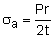where r is the inside radius and t is the wall thickness.Hoop Section Cut from
Cylindrical Vessel

In addition to the axial stress, there will be a hoop stress around the circumference. The hoop stress, σh, can be determined by taking a vertical hoop section that has a width of dx. The total horizontal pressure load pushing against the section will be p(2r dx) as shown in the diagram.

The top and bottom edge section will resist the pressure and exert a load of σh(t dx) (each edge). The edge loads have to equal the pressure load, or

p(2r dx) =σh(2t dx)

This can be simplified to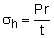where r is the inside radius and t is the wall thickness.

Spherical Pressure Vessel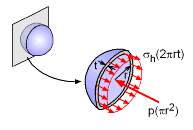Spherical Pressure Vessel
Cut in Half

A spherical pressure vessel is really just a special case of a cylinderical vessel. No matter how the a sphere is cut in half, the pressure load perpendicular to the cut must equal the shell stress load. This is the same situation with the axial direction in a cylindrical vessel. Equating the to loads give,

p(πr2) = σh(2πrt)

This can be simplified to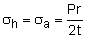Notice, the hoop and axial stress are the same due to symmetry.

Practice Homework and Test problems now available in the 'Eng Mechanics' mobile app
Includes over 400 problems with complete detailed solutions.
Available now at the Google Play Store and Apple App Store.